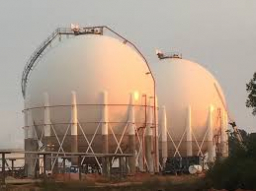# One gas

One gas tank was 2/3 full. The second tank of equal size was 1/5 full. The amount of gas in the 1st tank was 100 liters. How many liters is the amount of gas in the second tank?

V2 =  30 l

### Step-by-step explanation:Did you find an error or inaccuracy? Feel free to write us. Thank you!

Tips for related online calculators
Need help calculating sum, simplifying, or multiplying fractions? Try our fraction calculator.
Tip: Our volume units converter will help you convert volume units.